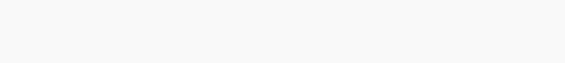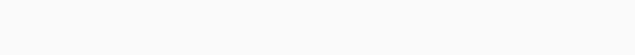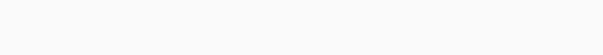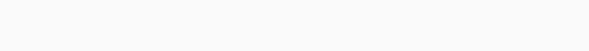# Program to find Unique Array Element

July 30, 2017
Posted by Abhi Andhariya

Programming and coding interview questions are an integral part of any Java, Python or C++ application developer interview. No matter on which language you have expertise, good problem solving skills shows how efficient you are in solving any problem.  In this post, we will discuss one of the very commonly asked programming question. .!!

## Problem Statement:

In a given array of integers, all the elements are duplicated except one element. Also elements are not sorted. Find the Unique Array Element in an optimal way.

For Example,In above array, all the elements except number 4 are duplicate. So output of our program should be 4.

There are many approaches to solve this problem.

## 1) Naive ApproachJava Program Implementation

```
/**
*
* @param inputArray
* @return returns unique Element in the array.
*         -1 if no unique element is available in the array.
*/
public static int naiveApproach(int[] inputArray)
{
Arrays.sort(inputArray);

int i=0;
while(i<inputArray.length)
{
if(inputArray[i]!=inputArray[i+1])
{
return inputArray[i];
}
i=i+2;
}

return -1;
}```

Time Complexity

Let's say , n is a size of input array.

1. Here, Time complexity of Arrays.sort() method is O(n logn) in worst case scenario.
2. Once array is sorted, traversing an array takes n/2 iterations.

So total time complexity of above algorithm is O(n logn + n/2)   i.e   O(n logn)

## 2) HashMap Approach

Instead of sorting an array, we will use HashMap to store the occurrence of each number.Java Program Implementation

```
/**
*
* @param inputArray
* @return returns unique Element in the array.
*         -1 if no unique element is available in the array.
*/
public static int hashMapApproach(int[] inputArray)
{
HashMap<Integer,Integer> map = new HashMap<>(inputArray.length);

for(int num : inputArray)
{
Integer occurrence = map.get(num);

// if occurrence is null, set it to 1,
// else increment the occurrence
map.put(num, occurrence==null ? 1 : occurrence+1);
}

// traversing entryset to find the key with occurrence == 1
for(Map.Entry<Integer, Integer> e : map.entrySet())
{
if(e.getValue() == 1)
{
return e.getKey();
}
}

return -1;
}```

Time Complexity

Let's say , n is a size of input array.

1. In best and average case, Time Complexity of HashMap insertion operation is O(1)  and in worst case it is O(n).
2. Once all the elements are inserted into HashMap, we need to traverse through  (Capacity of HashMap + size of HashMap)  elements of HashMap i.e O(capacity + n)

So total time complexity of above algorithm is O(n)

Space Complexity

In this approach, we are using an additional datastructure i.e. HashMap of size n. So space complexity of above program is O(n)

## 3) bit manipulation / XOR ApproachHow does this solution work?

For better understanding , we will use two basic properties of Bitwise XOR operation

1. XOR of any number with itself is zero. i.e n ^ n = 0

For Example, Binary of 5 is   (1 0 1)1. XOR is Commutative  i.e  x^y = y^x

For Example,  2 ^ 3 = 3 ^ 2

So for XOR of all the element of our array is ,Java Program Implementation

```
/**
*
* @param inputArray
* @return returns unique Element in the array.
*         -1 if no unique element is available in the array.
*/
public static int xorApproach(int[] inputArray)
{
int result = 0;
for(int i=0;i<inputArray.length;i++)
{
result ^= inputArray[i];
}

return (result>0 ? result : -1);
}```

Time Complexity

O(n)

Space Complexity

No extra space is required.

## Similar Java Programs

1. In a given array of duplicate integers, all the elements are duplicated even number of time. But one element is duplicated  odd number of times. Also elements are not sorted. Find the Array Element which is duplicated odd number of times in an optimal way. For Example,  output should be 4 for below array.We can solve this problem using HashMap and XOR Approaches.

In HashMap, approach we  need to tweak the logic in the entryset loop as below

```

for(Map.Entry<Integer, Integer> e : map.entrySet())
{
if(e.getValue() % 2 != 0)
{
return e.getKey();
}
}
```

In XOR Approach, we can use same function. 😉

1. You have given two integer arrays of size 5 and size 6 respectively. Both contains 5 equal elements (say 1 to 5), but second array contains one extra element. Both the arrays are unsorted. Find the extra element in second array.For Example, output should be 6 for below two arrays.We can solve this problem using HashMap and XOR Approaches. If we merge both the array, it will become the same problem which we have seen in this article. But instead of wasting our efforts into merging, we can traverse both the array independently.

1. In a given array of integers, all the elements are duplicated except one element. Elements are duplicated n number of times. Also elements are not sorted. Find the Unique Array Element in an optimal way. For Example, output should be 1 for below array.We can solve this problem using HashMap Approach.

That's all for this topic. If you guys have any suggestions or queries, feel free to drop a comment. We would be happy to add that in our post. You can also contribute your articles by creating contributor account here.

Happy Learning 🙂

If you like the content on CodePumpkin and if you wish to do something for the community and the planet Earth, you can donate to our campaign for planting more trees at CodePumpkin Cauvery Calling Campaign.

We may not get time to plant a tree, but we can definitely donate ₹42 per Tree.## abhi andhariya

Surviving Java Developer, Passionate Blogger, Table Tennis Lover, Bookworm, Occasional illustrator and a big fan of Joey Tribbiani, The Walking Dead and Game of Thrones...!!

Tags: , , ,

If you want someone to read your code, please put the code inside <pre><code> and </code></pre> tags. For example:
```<pre><code class="java">
String foo = "bar";
</code></pre>
```